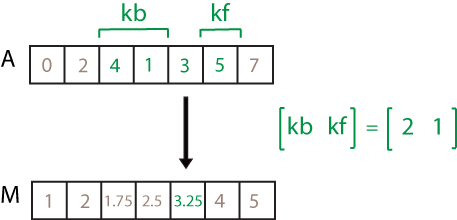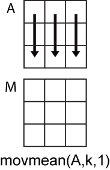# movmean

## 语法

``M = movmean(A,k)``
``M = movmean(A,[kb kf])``
``M = movmean(___,dim)``
``M = movmean(___,nanflag)``
``M = movmean(___,Name,Value)``

## 说明

``M = movmean(A,k)` 返回由局部 `k` 个数据点的均值组成的数组，其中每个均值是基于 `A` 的相邻元素的长度为 `k` 的滑动窗计算得出。当 `k` 为奇数时，窗口以当前位置的元素为中心。当 `k` 为偶数时，窗口以当前元素及其前一个元素为中心。当没有足够的元素填满窗口时，窗口将自动在端点处截断。当窗口被截断时，只根据窗口内的元素计算平均值。`M` 与 `A` 的大小相同。如果 `A` 是向量，`movmean` 将沿向量 `A` 的长度运算。如果 `A` 是多维数组，则 `movmean` 沿 `A` 的大小不等于 1 的第一个维度进行运算。`

``M = movmean(A,[kb kf])` 通过长度为 `kb+kf+1` 的窗口计算均值，其中包括当前位置的元素、后面的 `kb` 个元素和前面的 `kf` 个元素。`

``M = movmean(___,dim)` 为上述任一语法指定 `A` 的运算维度。例如，如果 `A` 是矩阵，则 `movmean(A,k,2)` 沿 `A` 的列运算，计算每行的 `k` 元素移动均值。`

``M = movmean(___,nanflag)` 指定在上述任意语法的计算中包括还是忽略 `NaN` 值。`movmean(A,k,'includenan')` 会在计算中包括所有 `NaN` 值，而 `movmean(A,k,'omitnan')` 则忽略这些值并基于较少的点计算均值。`

``M = movmean(___,Name,Value)` 使用一个或多个名称-值对组参数指定移动平均值的其他参数。例如，如果 `x` 是时间值向量，则 `movmean(A,k,'SamplePoints',x)` 相对于 `x` 中的时间计算移动平均值。`

## 示例

```A = [4 8 6 -1 -2 -3 -1 3 4 5]; M = movmean(A,3)```
```M = 1×10 6.0000 6.0000 4.3333 1.0000 -2.0000 -2.0000 -0.3333 2.0000 4.0000 4.5000 ```

```A = [4 8 6 -1 -2 -3 -1 3 4 5]; M = movmean(A,[2 0])```
```M = 1×10 4.0000 6.0000 6.0000 4.3333 1.0000 -2.0000 -2.0000 -0.3333 2.0000 4.0000 ```

`A = [4 8 6; -1 -2 -3; -1 3 4]`
```A = 3×3 4 8 6 -1 -2 -3 -1 3 4 ```
`M = movmean(A,3,2)`
```M = 3×3 6.0000 6.0000 7.0000 -1.5000 -2.0000 -2.5000 1.0000 2.0000 3.5000 ```

```A = [4 8 NaN -1 -2 -3 NaN 3 4 5]; M = movmean(A,3)```
```M = 1×10 6.0000 NaN NaN NaN -2.0000 NaN NaN NaN 4.0000 4.5000 ```

`M = movmean(A,3,'omitnan')`
```M = 1×10 6.0000 6.0000 3.5000 -1.5000 -2.0000 -2.5000 0 3.5000 4.0000 4.5000 ```

```A = [4 8 6 -1 -2 -3]; k = hours(3); t = datetime(2016,1,1,0,0,0) + hours(0:5)```
```t = 1x6 datetime Columns 1 through 3 01-Jan-2016 00:00:00 01-Jan-2016 01:00:00 01-Jan-2016 02:00:00 Columns 4 through 6 01-Jan-2016 03:00:00 01-Jan-2016 04:00:00 01-Jan-2016 05:00:00 ```
`M = movmean(A,k,'SamplePoints',t)`
```M = 1×6 6.0000 6.0000 4.3333 1.0000 -2.0000 -2.5000 ```

```A = [4 8 6 -1 -2 -3 -1 3 4 5]; M = movmean(A,3,'Endpoints','discard')```
```M = 1×8 6.0000 4.3333 1.0000 -2.0000 -2.0000 -0.3333 2.0000 4.0000 ```

## 输入参数• `movmean(A,k,1)``A` 的每列计算包含 `k` 个元素的移动均值，并返回一个 `m`×`n` 矩阵。• `movmean(A,k,2)``A` 的每行计算包含 `k` 个元素的移动均值，并返回一个 `m`×`n` 矩阵。`NaN` 条件，指定为下列值之一：

• `'includenan'` - 计算均值时包括输入中的 `NaN` 值，其对应输出为 `NaN` 值。

• `'omitnan'` - 忽略输入中的所有 `NaN` 值。如果窗口只包含 `NaN` 值，则 `movmean` 返回 `NaN`

### 名称-值参数

`'Endpoints'`描述
`'shrink'`在窗口接近输入端点时缩小窗口的大小，从而只包括现有元素。
`'discard'`当窗口不与现有元素完全重叠时，不输出任何平均值。
`'fill'`将不存在的元素替换为 `NaN`

## 详细信息

### 均值

`$\mu =\frac{1}{N}\sum _{i=1}^{N}{A}_{i}.$`